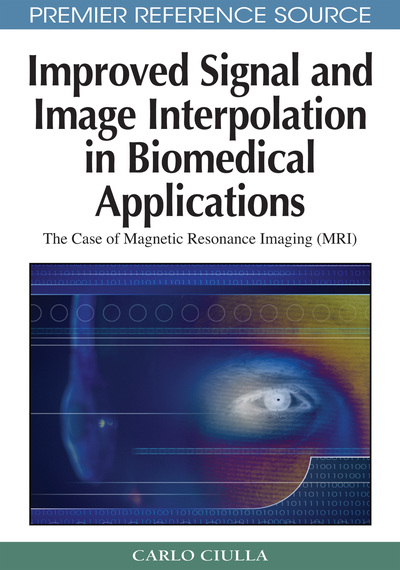# Assessment of the Truth Foreseen in the Intuition

Carlo Ciulla (Lane College, USA)
DOI: 10.4018/978-1-60566-202-2.ch005
Available
\$37.50
No Current Special Offers

## Abstract

This chapter continues the investigation conducted through deduction from the previous three chapters. Chapter II of the book has brought to light an intuition that has allowed the conception of the Sub- Pixel Efficacy Region and its definition. Chapter III outlines the conception of the Intensity-Curvature functional as a measure of the change in energy level of the signal because of the effect of the model interpolation function on the signal (image). Chapter IV studies the Intensity-Curvature Functional (?E) and through the solution of the polynomial system obtained from the first order partial derivatives of ?E, elucidates that a spatial set of points within the voxel is calculated from the Intensity-Curvature Functional and is assigned the name of Sub-pixel Efficacy Region (SRE).
Chapter Preview
Top

## Introduction

This chapter continues the investigation conducted through deduction from the previous three chapters. Chapter II of the book has brought to light an intuition that has allowed the conception of the Sub-Pixel Efficacy Region and its definition. Chapter III outlines the conception of the Intensity-Curvature functional as a measure of the change in energy level of the signal because of the effect of the model interpolation function on the signal (image). Chapter IV studies the Intensity-Curvature Functional (ΔE) and through the solution of the polynomial system obtained from the first order partial derivatives of ΔE, elucidates that a spatial set of points within the voxel is calculated from the Intensity-Curvature Functional and is assigned the name of Sub-pixel Efficacy Region (SRE).

The next step is then to assess the truth foreseen in the intuition of Chapter II for what concerns the definition of the Sub-pixel Efficacy Region. The definition of the SRE given in Chapter II has to be re-evaluated on the basis of the results of Chapter IV.

Conceptually, what Chapter II states is that there is a region within the voxel where the value of the second order partial derivative (curvature) of the model interpolation function is bounded by two constants. Also, Chapter II tentatively asserts that within this region the model interpolation function has optimal approximation characteristics. That means that the function admits minimal interpolation error with respect to the error obtainable in the spatial domain that is located outside the SRE.

On the basis of the theoretical grounds furnished in Chapter III as far as the meaning of the Intensity-Curvature Functional is concerned, Chapter IV derives the spatial set of points within the voxel where the extremes of ΔE are located and assigns to these points the name: Sub-pixel Efficacy Region.

Formulas given for the SRE in Chapter IV (equations (16), (17) and (18)) do not have immediate correspondence to the definition of the SRE given in Chapter II.

Therefore, it is due to investigate what is the degree of match between the two definitions of the SRE: (i) the one given in Chapter II, which is purely theoretical, and (ii) the other one deducted through the study of the Intensity-Curvature Functional in Chapter IV, which is practical. Through the evaluation of the degree of match between these two definitions of the SRE it is possible to assess on the truth that was foreseen in the intuition presented in Chapter II.

### THE ASSESSMENT

It is presented here and in appendix III the mathematical process that through further study of equations (16), (17) and (18) of Chapter IV attempts to establish as to whether or not the SRE set of points found in Chapter IV has the theoretical property stated in Chapter II. This means to see as to if at the SRE spatial points of Chapter IV the local curvature of the model interpolation function is bounded by the constants given by the equations (5) through (10) of Chapter II. In order to undertake the investigative process and for convenience to the reader let us recall the definition of SRE given in Chapters II and IV.

### Chapter II

(x, y, z) belongs to the SRE if and only if the following is true: z ≤ ωf bxy / (ωf2 - bxy) (1) z ≥ ωf bxy / (ωf2 + bxy) (2) y ≤ ωf bxz / (ωf2 - bxz) (3) y ≥ ωf bxz / (ωf2 + bxz) (4) x ≤ ωf byz / (ωf2 - byz) (5) x ≥ ωf byz / (ωf2 + byz) (6)

## Complete Chapter List

Search this Book:
Reset